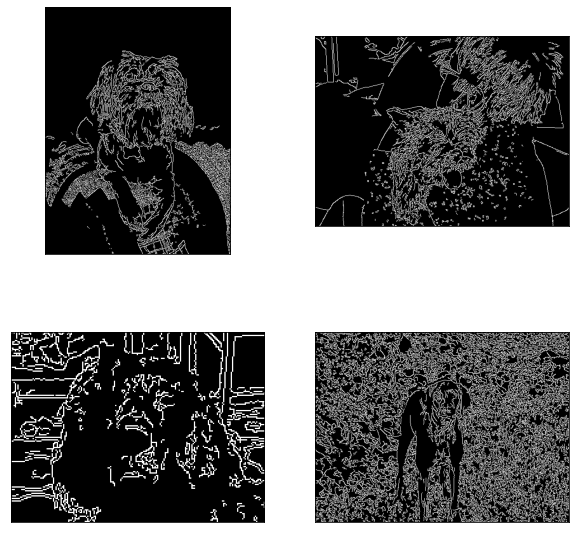List Examples¶

:
from easycv import List, Image
from easycv.transforms import GrayScale, Canny

Creating a list¶

We can create a list of random images using random()

:
l = List.random(4)

To view the images, we can call the show method

:
l.show()Apply transforms¶

We can apply transforms to List exactly like we would apply them to a single image

:
l = l.apply(GrayScale())
l.show()Access single images¶

Lists support indexing/slicing like normal python lists

:
l
::
l[2:4].show()Parallel processing¶

To process images in parallel just set parallel to True on apply. Note that for non-lazy images, small ammounts of images and fast operations this could be slower thatn withou parallelism!

:
l = l.apply(Canny(), parallel=True)
l.show()